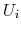Madagascar Programming Reference ManualNext: C program Up: An example: Finite-Difference modeling Previous: An example: Finite-Difference modeling

# Introduction

This section presents time-domain finite-difference modeling  written with the RSF library. The program is demonstrated with the C, C++ and Fortran 90 interfaces. The acoustic wave-equation

 can be written aswhereis the Laplacian symbol,is the source wavelet,is the velocity, andis a scalar wavefield. A discrete time-step involves the following computations:where,andrepresent the propagating wavefield at various time steps. In this exercise we shall use a discrete Laplacian accurate up to the fourth order and the second derivative of time is accurate up to the second order.

2011-07-02